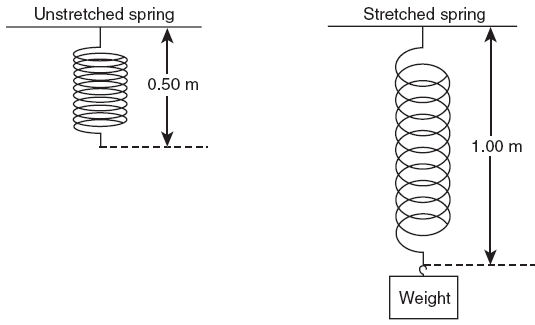﻿ Elastic PE and K NotesTry this at home!

Egg trick learn how now

PE and k

e) PEs in terms of k - Ideal Spring

1. P.E.s = Fx

Average Force:  F = F/2

2.  PE = (F/2)x

substitute  F = Kx (On reference)

3.  P.E.s = (1/2)(Kx)x

 P.E.s = 1/2(kx2)

(On reference)

 Ex 1) A force of 12. N stretches a spring and makes it .15m longer   a) What the spring constant (k) of this spring?Ex 1) A force of 12. N stretches a spring and makes it .15m longer

a) What's the spring constant (k) of this spring?

k = F/x

= 12. N/.15 m

= 80. N/m

b) P.E. of this spring?

PEs = (½)Kx2

= 1/2(80. N/m)(.15 m2)

Be sure to use parenthesis when calculating!!

PEs = .90 JoulesEx 2) If 15. joules of energy are stored in the stretched spring below, what is the value of the spring constant?Ex 2) If 15. joules of energy are stored in the stretched spring, what is the value of the spring constantP.E.s = 1/2(kx2)

15. J = (½)k(.50 m2)

1/2 in decimal form is .50

15. J = .50 k(.25)

15  = .125 k

Divide both sides by .125

k = 120 N/mWhat's wrong with this picture?   P.E.s = (1/2)kx2 x = elongationP.E.s = 1/2(kx2) PE, X direct square Plot should be curved

W = DPE

29) A 1200 kg car rolling on a horizontal surface has a v = 65 km/hr when it strikes a horizontal coiled spring and is brought to rest in a distance of 2.2 m.

What is the spring constant of the spring?

DKE = DPE

65 km/hr x [1000m/km][1hr/3600]

= 18 m/s

1/2mv2 = 1/2kx2

mv2 = kx2

mv2/[x2] = k

8.1 x 104 N/m